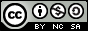- 目录 -
LeetCode 491 递增子序列(枚举子集)

输入: [4, 6, 7, 7]



1. 给定数组的长度不会超过15。
2. 数组中的整数范围是 [-100,100]。
3. 给定数组中可能包含重复数字，相等的数字应该被视为递增的一种情况。

## 思路

• 这个序列是不是一个递增序列
• 去重

## 代码

  1 2 3 4 5 6 7 8 9 10 11 12 13 14 15 16 17 18 19 20 21 22 23 24 25 26 27 28 29 30 31 32 33 34 35 36 37  class Solution { public: vector> findSubsequences(vector &nums) { vector> ans; unordered_map mp; int n = nums.size(); if (!n) return ans; vector tmp; for (int i = 0; i < (1 << n); i++) { tmp.clear(); string s = ""; int t = -200, flag = 1; for (int j = 0; j < n; j++) if (i & (1 << j)) { if (nums[j] < t) { flag = 0; break; } t = nums[j]; tmp.push_back(nums[j]); s = s + to_string(nums[j]) + "|"; } if (flag && tmp.size() >= 2 && !mp[s]) { ans.push_back(tmp); mp[s]++; } } return ans; } };